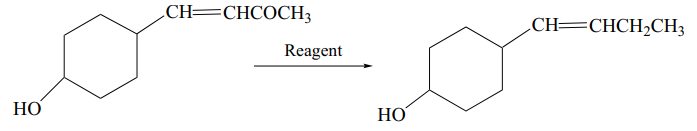# In the given transformation, which of the following is the most appropriate reagent?1. A

${\mathrm{NH}}_{2}{\mathrm{NH}}_{2},{\mathrm{OH}}^{-}$

2. B

$\mathrm{Zn}-\mathrm{Hg}/\mathrm{HCl}$

3. C

4. D

${\mathrm{NaBH}}_{4}$

FREE Lve Classes, PDFs, Solved Questions, PYQ's, Mock Tests, Practice Tests, and Test Series!

+91

Verify OTP Code (required)

I agree to the terms and conditions and privacy policy.

### Solution:

The Wolff-Kishner reduction (which uses hydrazine,  and a strong base ${\mathrm{OH}}^{-}\right)$ reduces only  group without affecting the $-\mathrm{C}=\mathrm{C}-$ group.

## Related content

Join Infinity Learn Regular Class Program!

Sign up & Get instant access to FREE PDF's, solved questions, Previous Year Papers, Quizzes and Puzzles!

+91

Verify OTP Code (required)

I agree to the terms and conditions and privacy policy.